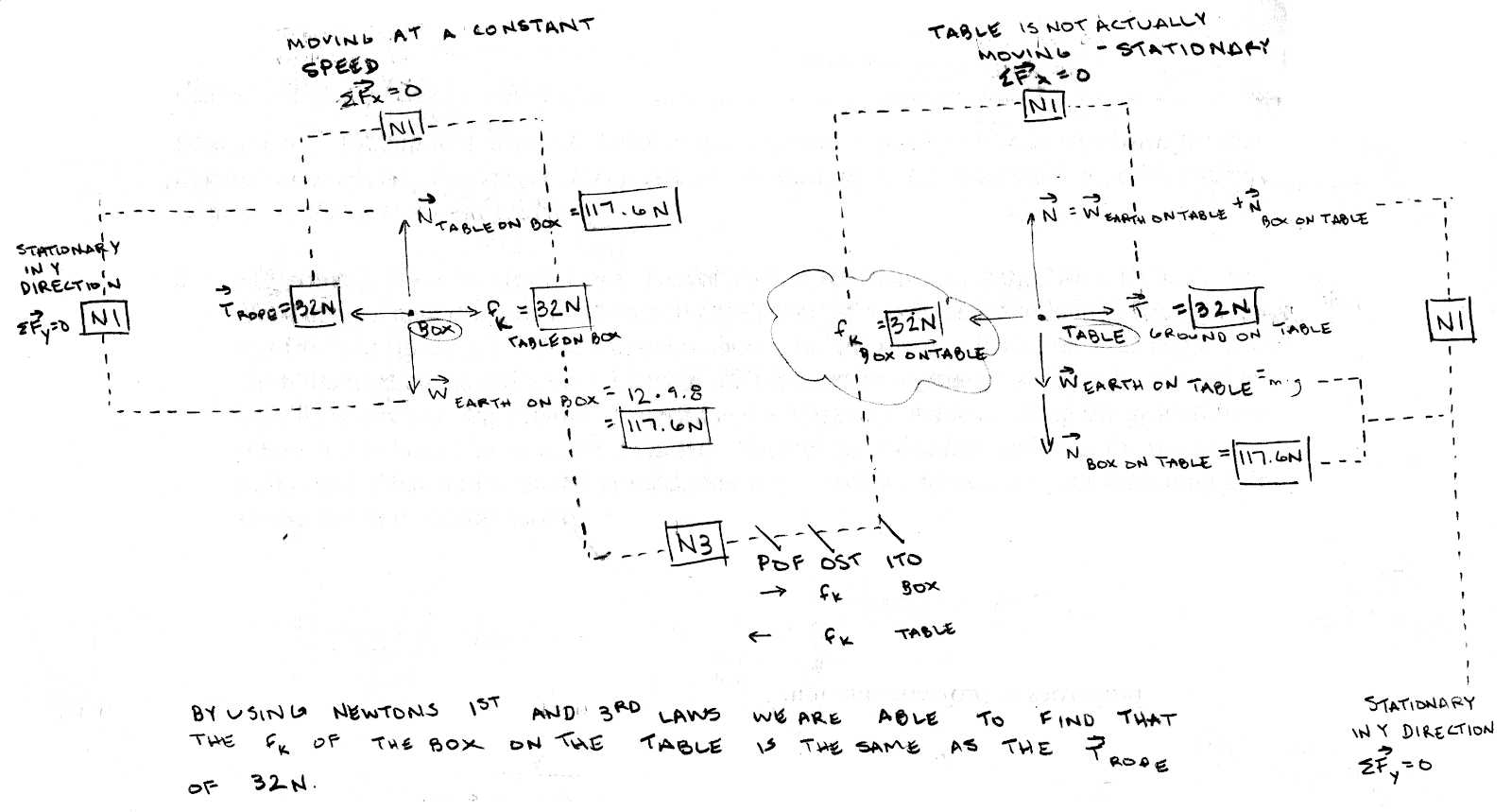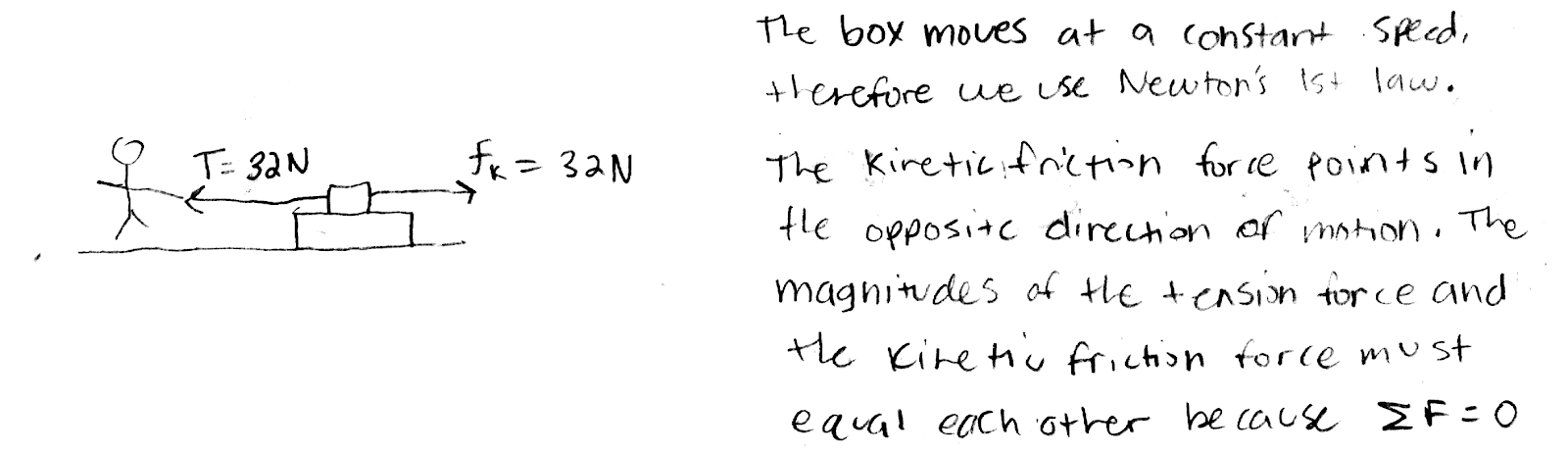## 20191011

### Physics midterm question: pulled box pulling on table underneath

Physics 205A Midterm 1, fall semester 2019
Cuesta College, San Luis Obispo, CAA Physics 205A student applies a force (magnitude of 32 N, directed to the left) to pull on a rope attached to a 12.0 kg box, which moves at constant speed to the left across a fixed, stationary table. Discuss why both the magnitude and direction of the kinetic friction force of the box on the table would also be 32 N, directed to the left. Explain your reasoning using free-body diagram(s), the properties of forces, and Newton's laws.

• p:
Correct. Complete free-body diagram(s), and discusses/demonstrates:
1. the box has two horizontal forces acting on it:
Tension force T of student on box (32 N, to the left),
Kinetic friction force  fk of table on box (32 N, to the right),
and since the box has a constant velocity ("constant speed to the left"), these two horizontal forces must be equal in magnitude due to Newton's first law; and

2. from Newton's third law, these two forces must have equal magnitudes and opposite directions:
Kinetic friction force  fk of table on box (32 N, to the right),
Kinetic friction force  fk of box on table (32 N, to the left).
• r:
As (p), but argument indirectly, weakly, or only by definition supports the statement to be proven, or has minor inconsistencies or loopholes.
• t:
Nearly correct, but argument has conceptual errors, or is incomplete. Some substantive attempt at analyzing forces using Newton's laws and free-body diagrams. Typically discusses Newton's first law for the forces acting on the box, but subsequent discussion of Newton's third law is omitted or merely implied.
• v:
Limited relevant discussion of supporting evidence of at least some merit, but in an inconsistent or unclear manner. Some garbled attempt at using Newton's laws and free-body diagrams.
• x:
Implementation of ideas, but credit given for effort rather than merit. No systematic attempt at using Newton's laws and free-body diagrams.
• y:
Irrelevant discussion/effectively blank.
• z:
Blank.
Sections 70854, 70855
Exam code: midterm01duCk
p: 15 students
r: 5 students
t: 14 students
v: 15 students
x: 2 students
y: 0 students
z: 0 students

A sample "p" response (from student 6900):Another sample "p" response (from student 2533; note that the static friction force of the ground on table pointing to the right is denoted as a tension force):A sample "t" response (from student 1995), discussing Newton's first law for the box, demonstrating that the kinetic friction force of the table on the box points to the right with a magnitude of 32 N: